International
Tables for
Crystallography
Volume F
Crystallography of biological macromolecues
Edited by M. G. Rossmann and E. Arnold

International Tables for Crystallography (2006). Vol. F, ch. 22.3, pp. 553-555   | 1 | 2 |

## Section 22.3.2. Theory

K. A. Sharpa*

aE. R. Johnson Research Foundation, Department of Biochemistry and Biophysics, University of Pennsylvania, Philadelphia, PA 19104-6059, USA
Correspondence e-mail: sharp@crystal.med.upenn.edu

### 22.3.2. Theory

| top | pdf |

#### 22.3.2.1. The response of the system to electrostatic fields

| top | pdf |

The response to the electrostatic field arising from the molecular charge distribution arises from three physical processes: electronic polarization, reorientation of permanent dipolar groups and redistribution of mobile ions in the solvent. Movement of ionized side chains, if significant, is sometimes viewed as part of the dielectric response of the protein, and sometimes explicitly as a conformational change of the molecule.

Electronic polarizability can be represented either by point inducible dipoles (Warshel & Åqvist, 1991) or by a dielectric constant. The latter approach relates the electrostatic polarization, P(r) (the mean dipole moment induced in some small volume V) to the Maxwell (total) field, E(r), and the local dielectric constant representing the response of that volume,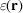, according to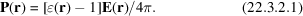The contribution of electronic polarizability to the dielectric constant of most organic material and water is fairly similar. It can be evaluated by high-frequency dielectric measurements or the refractive index, and it is in the range 2–2.5.

The reorientation of groups such as the peptide bond or surrounding water molecules which have large permanent dipoles is an important part of the overall response. This response too may be treated using a dielectric constant, i.e. using equation (22.3.2.1) with a larger value of the dielectric constant that incorporates the additional polarization from dipole reorientation. An alternative approach to equation (22.3.2.1) for treating the dipole reorientation contribution of water surrounding the macromolecules is the Langevin dipole model (Lee et al., 1993; Warshel & Åqvist, 1991; Warshel & Russell, 1984). Four factors determine the degree of response from permanent dipoles: (i) the dipole-moment magnitude; (ii) the density of such groups in the protein or solvent; (iii) the freedom of such groups to reorient; and (iv) the degree of cooperativity between dipole motions. Thus, water has a high dielectric constant (= 78.6 at 25 °C). For electrostatic models based on dielectric theory, the experimental solvent dielectric constant, reflecting the contribution of electronic polarizability and dipole reorientation, is usually used. From consideration of the four factors that determine the dielectric response, macromolecules would be expected to have a much lower dielectric constant than the solvent. Indeed, theoretical studies of the dielectric behaviour of amorphous protein solids (Gilson & Honig, 1986; Nakamura et al., 1988) and the interior of proteins in solution (Simonson & Brooks, 1996; Simonson & Perahia, 1995; Smith et al., 1993), and experimental measurements (Takashima & Schwan, 1965) provide an estimate of= 2.5–4 for the contribution of dipolar groups to the protein dielectric.

The Langevin model can account for the saturation of the response at high fields that occurs if the dipoles become highly aligned with the field. The dielectric model can also be extended to incorporate saturation effects (Warwicker, 1994), although there is a compensating effect of electrostriction, which increases the local dipole density (Jayaram, Fine et al., 1989). While the importance of saturation effects would vary from case to case, linear solvent dielectric models have proven sufficiently accurate for most protein applications to date.

Charge groups on molecules will attract solvent counter-ions and repel co-ions. The most common way of treating this charge rearrangement is via the Boltzmann model, where the net charge density of mobile ions is given by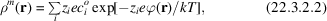where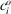is the bulk concentration of an ion of type i, valence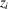, andis the average potential (an approximation to the potential of mean force) at position r. The Boltzmann approach neglects the effect of ion size and correlations between ion positions. Other models for the mobile-ion behaviour that account for these effects are integral equation models and MC models (Bacquet & Rossky, 1984; Murthy et al., 1985; Olmsted et al., 1989, 1991; Record et al., 1990). These studies show that ion size and correlation effects do not compromise the Boltzmann model significantly for monovalent (1–1) salts at mid-range concentrations 0.001–0.5 M, and consequently it is widely used for modelling salt effects in proteins and nucleic acids.

#### 22.3.2.2. Dependence of the potential on the charge distribution

| top | pdf |

The potential at a point in space, r, arising from some charge density distribution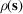and some dipole density distribution P(s) (which includes polarization) is given by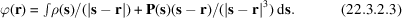The total charge distribution is the sum of the explicit charge distribution on the molecule and that from the mobile solvent ion distribution,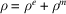. Substituting for the dielectric polarization using equation (22.3.2.1) and for the mobile ion charge distribution using equation (22.3.2.2), the potential may be expressed in terms of a partial differential equation, the Poisson–Boltzmann (PB) equation: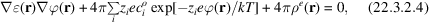which relates the potential, molecular charge and dielectric distributions,,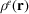and, respectively. Contributions to the polarizability from electrons, a molecule's permanent dipoles and solvent dipoles are incorporated into this model by using an appropriate value for the dielectric for each region of protein and solvent. Values for protein atomic charges, radii and dielectric constants suitable for use with the Poisson–Boltzmann equation are available in the literature (Jean-Charles et al., 1990; Mohan et al., 1992; Simonson & Brünger, 1994; Sitkoff et al., 1994). For protein applications, the Boltzmann term in equation (22.3.2.4) is usually linearized to become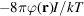where I is the ionic strength, whereas for nucleic acids and molecules of similarly high charge density the full nonlinear equation is used.

#### 22.3.2.3. The concepts of screening, reaction potentials, solvation, dielectric, polarity and polarizability

| top | pdf |

Application of a classical electrostatic view to macromolecular electrostatics involves a number of useful concepts that describe the physical behaviour. It should first be recognized that the potential at a particular charged atom i includes three physically distinct contributions. The first is the direct or Coulombic potential of j at i. The second is the potential at i generated by the polarization (of a molecule, water and ion atmosphere) induced by j. This is often referred to as the screening potential, since it opposes the direct Coulombic potential. The third arises from the polarization induced by i itself. This is often referred to as the reaction or self-potential, or if solvent is involved, as the solvation potential.

When using models that apply the concept of a dielectric constant (a measure of polarizability) to a macromolecule, it is important to distinguish between polarity and polarizability. Briefly, polarity may be thought of as describing the density of charged and dipolar groups in a particular region. Polarizability, by contrast, refers to the potential for reorganizing charges, orienting dipoles and inducing dipoles. Thus polarizability depends both on the polarity and the freedom of dipoles to reorganize in response to an applied electric field. When a protein is folding or undergoing a large conformational rearrangement, the peptide groups may be quite free to reorient. In the folded protein, these may become spatially organized so as to stabilize another charge or dipole, creating a region with high polarity, but with low polarizability, since there is much less ability to reorient the dipolar groups in response to a new charge or dipole without significant disruption of the structure. Thus, while there is still some discussion about the value and applicability of a protein dielectric constant, it is generally agreed that the interior of a macromolecule is a less polarizable environment compared to solvent. This difference in polarizability has a significant effect on the potential distribution.

Formally charged groups on proteins, particularly the longer side chains on the surface of proteins, Arg, Lys, and to a lesser extent Glu and Asp, have the ability to alter their conformation in response to electrostatic fields. In addition, information about fluctuations about their mean position may need to be included in calculating average properties. Three approaches to modelling protein formal charge movements can be taken. The first is to treat the motions within the dielectric response. In this approach, the protein may be viewed as having a dielectric higher than 2.5–4 in the regions of these charged groups, particularly at the surface, where the concentration and mobility of these groups may give an effective dielectric of 20 or more (Antosiewicz et al., 1994; Simonson & Perahia, 1995; Smith et al., 1993). A second approach is to model the effect of charge motions on the electrostatic quantity of interest explicitly, e.g. with MD simulations (Langsetmo et al., 1991; Wendoloski & Matthew, 1989). This involves generating an ensemble of structures with different atomic charge distributions. The third approach is based on the fact that one is often interested in a specific biological process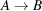in which one can evaluate the structure of the protein in states A and B (experimentally or by modelling), and any change in average charge positions is incorporated at the level of different average explicit charge distribution inputs for the calculation, modelling only the electronic, dipolar and salt contributions as the response.

The term effective' dielectric constant is sometimes used in the literature to describe the strength of interaction between two charges,and. This is defined as the ratio of the observed or calculated interaction strength, U, to that expected between the same two charges in a vacuum: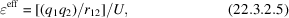where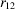is the distance between the charges. If the system were completely homogeneous in terms of its electrostatic response and involved no charge rearrangement then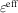would describe the dielectric constant of the medium containing the charges. This is generally never the case: the strength of interaction in a protein system is determined by the net contribution from protein, solvent and ions, sodoes not give information about the dielectric property of any particular region of space. In fact, in the same system different charge–charge interactions will generally yield different values of. Thusis really no more than its definition – a measure of the strength of interaction – and it cannot be used directly to answer questions about the protein dielectric constant, for example. Rather, it is one of the quantities that one aims to extract from theoretical models to compare with an experiment.

#### 22.3.2.4. Calculation of energies and forces

| top | pdf |

Once the electrostatic potential distribution has been obtained, calculation of experimental properties usually requires evaluation of the electrostatic energy or force. For a linear system (where the dielectric and ionic responses are linear) the electrostatic free energy is given by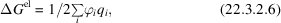where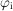is the potential at an atom with charge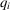. The most common source of nonlinearity is the Boltzmann term in the PB equation (22.3.2.4) for highly charged molecules such as nucleic acids. The total electrostatic energy in this case is (Reiner & Radke, 1990; Sharp & Honig, 1990; Zhou, 1994)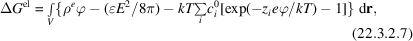where the integration is now over all space.

The general expression for the electrostatic force on a charge q is given by the gradient of the total free energy with respect to that charge's position,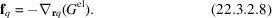If the movement of that charge does not affect the potential distribution due to the other charges and dipoles, then equation (22.3.2.8) can be evaluated using the test charge' approach, in which case the force depends only on the gradient of the potential or the field at the charge: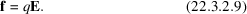However, in a system like a macromolecule in water, which has a non-homogeneous dielectric, forces arise between a charge and any dielectric boundary due to image charge (reaction potential) effects. A similar effect to the `dielectric pressure' force arises from solvent-ion pressure at the solute–solvent boundary. This results in a force acting to increase the solvent exposure of charged and polar atoms. An expression for the force that includes these effects has been derived within the PB model (Gilson et al., 1993):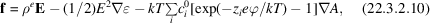where A is a function describing the accessibility to solvent ions, which is 0 inside the protein, and 1 in the solvent, and whose gradient is nonzero only at the solute–solvent surface. Similarly, in a two-dielectric model (solvent plus molecule) the gradient ofis nonzero only at the molecular surface. The first term accounts for the force acting on a charge due to a field, as in equation (22.3.2.9), while the second and third terms account for the dielectric surface pressure and ionic atmosphere pressure terms respectively. Equation (22.3.2.10) has been used to combine the PB equation and molecular mechanics (Gilson et al., 1995).

#### 22.3.2.5. Numerical methods

| top | pdf |

A variety of numerical methods exist for calculating electrostatic potentials of macromolecules. These include numerical solution of self-consistent field electrostatic equations, which has been used in conjunction with the protein dipole–Langevin dipole method (Lee et al., 1993). Numerical solution of the Poisson–Boltzmann equation requires the solution of a three-dimensional partial differential equation, which can be nonlinear. Many numerical techniques, some developed in engineering fields to solve differential equations, have been applied to the PB equation. These include finite-difference methods (Bruccoleri et al., 1996; Gilson et al., 1988; Nicholls & Honig, 1991; Warwicker & Watson, 1982), finite-element methods (Rashin, 1990; Yoon & Lenhoff, 1992; Zauhar & Morgan, 1985), multigridding (Holst & Saied, 1993; Oberoi & Allewell, 1993), conjugate-gradient methods (Davis & McCammon, 1989) and fast multipole methods (Bharadwaj et al., 1994; Davis, 1994). Methods for treating the nonlinear PB equation include under-relaxation (Jayaram, Sharp & Honig, 1989) and powerful inexact Newton methods (Holst et al., 1994). The nonlinear PB equation can also be solved via a self-consistent field approach, in which one calculates the potential using equation (22.3.2.5), then the mobile charge density is calculated using equation (22.3.2.3), and the procedure is repeated until convergence is reached (Pack & Klein, 1984; Pack et al., 1986). The method allows one to include more elaborate models for the ion distribution, for example incorporating the finite size of the ions (Pack et al., 1993). Approximate methods based on spherical approximations (Born-type models) have also been used (Schaeffer & Frommel, 1990; Still et al., 1990). Considerable numerical progress has been made in finite methods, and accurate rapid algorithms are available. The reader is referred to the original references for numerical details.

### References

Antosiewicz, J., McCammon, J. A. & Gilson, M. K. (1994). Prediction of pH-dependent properties of proteins. J. Mol. Biol. 238, 415–436.Google Scholar
Bacquet, R. & Rossky, P. (1984). Ionic atmosphere of rodlike polyelectrolytes. A hypernetted chain study. J. Phys. Chem. 88, 2660.Google Scholar
Bharadwaj, R., Windemuth, A., Sridharan, S., Honig, B. & Nicholls, A. (1994). The fast multipole boundary-element method for molecular electrostatics – an optimal approach for large systems. J. Comput. Chem. 16, 898–913.Google Scholar
Bruccoleri, R. E., Novotny, J., Sharp, K. A. & Davis, M. E. (1996). Finite difference Poisson–Boltzmann electrostatic calculations: increased accuracy achieved by harmonic dielectric smoothing and charge anti-aliasing. J. Comput. Chem. 18, 268–276.Google Scholar
Davis, M. E. (1994). The inducible multipole solvation model – a new model for solvation effects on solute electrostatics. J. Chem. Phys. 100, 5149–5159.Google Scholar
Davis, M. E. & McCammon, J. A. (1989). Solving the finite difference linear Poisson Boltzmann equation: comparison of relaxational and conjugate gradient methods. J. Comput. Chem. 10, 386–395.Google Scholar
Gilson, M., Davis, M., Luty, B. & McCammon, J. (1993). Computation of electrostatic forces on solvated molecules using the Poisson–Boltzmann equation. J. Phys. Chem. 97, 3591–3600.Google Scholar
Gilson, M. & Honig, B. (1986). The dielectric constant of a folded protein. Biopolymers, 25, 2097–2119.Google Scholar
Gilson, M., McCammon, J. & Madura, J. (1995). Molecular dynamics simulation with continuum solvent. J. Comput. Chem. 16, 1081–1095.Google Scholar
Gilson, M., Sharp, K. A. & Honig, B. (1988). Calculating the electrostatic potential of molecules in solution: method and error assessment. J. Comput. Chem. 9, 327–335.Google Scholar
Holst, M., Kozack, R., Saied, F. & Subramaniam, S. (1994). Protein electrostatics – rapid multigrid-based Newton algorithm for solution of the full nonlinear Poisson–Boltzmann equation. J. Biomol. Struct. Dyn. 11, 1437–1445.Google Scholar
Holst, M. & Saied, F. (1993). Multigrid solution of the Poisson–Boltzmann equation. J. Comput. Chem. 14, 105–113.Google Scholar
Jayaram, B., Fine, R., Sharp, K. A. & Honig, B. (1989). Free energy calculations of ion hydration: an analysis of the Born model in terms of microscopic simulations. J. Phys. Chem. 93, 4320–4327.Google Scholar
Jayaram, B., Sharp, K. A. & Honig, B. (1989). The electrostatic potential of B-DNA. Biopolymers, 28, 975–993.Google Scholar
Jean-Charles, A., Nicholls, A., Sharp, K., Honig, B., Tempczyk, A., Hendrickson, T. & Still, C. (1990). Electrostatic contributions to solvation energies: comparison of free energy perturbation and continuum calculations. J. Am. Chem. Soc. 113, 1454–1455.Google Scholar
Langsetmo, K., Fuchs, J. A., Woodward, C. & Sharp, K. A. (1991). Linkage of thioredoxin stability to titration of ionizable groups with perturbed pKa. Biochemistry, 30, 7609–7614.Google Scholar
Lee, F., Chu, Z. & Warshel, A. (1993). Microscopic and semimicroscopic calculations of electrostatic energies in proteins by the Polaris and Enzymix programs. J. Comput. Chem. 14, 161–185.Google Scholar
Mohan, V., Davis, M. E., McCammon, J. A. & Pettitt, B. M. (1992). Continuum model calculations of solvation free energies – accurate evaluation of electrostatic contributions. J. Phys. Chem. 96, 6428–6431.Google Scholar
Murthy, C. S., Bacquet, R. J. & Rossky, P. J. (1985). Ionic distributions near polyelectrolytes. A comparison of theoretical approaches. J. Phys. Chem. 89, 701.Google Scholar
Nakamura, H., Sakamoto, T. & Wada, A. (1988). A theoretical study of the dielectric constant of a protein. Protein Eng. 2, 177–183.Google Scholar
Nicholls, A. & Honig, B. (1991). A rapid finite difference algorithm utilizing successive over-relaxation to solve the Poisson–Boltzmann equation. J. Comput. Chem. 12, 435–445.Google Scholar
Oberoi, H. & Allewell, N. (1993). Multigrid solution of the nonlinear Poisson–Boltzmann equation and calculation of titration curves. Biophys. J. 65, 48–55.Google Scholar
Olmsted, M. C., Anderson, C. F. & Record, M. T. (1989). Monte Carlo description of oligoelectrolyte properties of DNA oligomers. Proc. Natl Acad. Sci. USA, 86, 7766–7770.Google Scholar
Olmsted, M. C., Anderson, C. F. & Record, M. T. (1991). Importance of oligoelectrolyte end effects for the thermodynamics of conformational transitions of nucleic acid oligomers. Biopolymers, 31, 1593–1604.Google Scholar
Pack, G., Garrett, G., Wong, L. & Lamm, G. (1993). The effect of a variable dielectric coefficient and finite ion size on Poisson–Boltzmann calculations of DNA–electrolyte systems. Biophys. J. 65, 1363–1370.Google Scholar
Pack, G. R. & Klein, B. J. (1984). The distribution of electrolyte ions around the B- and Z-conformers of DNA. Biopolymers, 23, 2801.Google Scholar
Pack, G. R., Wong, L. & Prasad, C. V. (1986). Counterion distribution around DNA. Nucleic Acids Res. 14, 1479.Google Scholar
Rashin, A. A. (1990). Hydration phenomena, classical electrostatics and the boundary element method. J. Phys. Chem. 94, 725–733.Google Scholar
Record, T., Olmsted, M. & Anderson, C. (1990). Theoretical studies of the thermodynamics of ion interaction with DNA. In Theoretical biochemistry and molecular biophysics. New York: Adenine Press.Google Scholar
Reiner, E. S. & Radke, C. J. (1990). Variational approach to the electrostatic free energy in charged colloidal suspensions. J. Chem. Soc. Faraday Trans. 86, 3901.Google Scholar
Schaeffer, M. & Frommel, C. (1990). A precise analytical method for calculating the electrostatic energy of macromolecules in aqueous solution. J. Mol. Biol. 216, 1045–1066.Google Scholar
Sharp, K. & Honig, B. (1990). Calculating total electrostatic energies with the non-linear Poisson–Boltzmann equation. J. Phys. Chem. 94, 7684–7692.Google Scholar
Simonson, T. & Brooks, C. L. (1996). Charge screening and the dielectric-constant of proteins: insights from molecular-dynamics. J. Am. Chem. Soc. 118, 8452–8458.Google Scholar
Simonson, T. & Brünger, A. (1994). Solvation free energies estimated from a macroscopic continuum theory. J. Phys. Chem. 98, 4683–4694.Google Scholar
Simonson, T. & Perahia, D. (1995). Internal and interfacial dielectric properties of cytochrome c from molecular dynamics in aqueous solution. Proc. Natl Acad. Sci. USA, 92, 1082–1086.Google Scholar
Sitkoff, D., Sharp, K. & Honig, B. (1994). Accurate calculation of hydration free energies using macroscopic solvent models. J. Phys. Chem. 98, 1978–1988.Google Scholar
Smith, P., Brunne, R., Mark, A. & van Gunsteren, W. (1993). Dielectric properties of trypsin inhibitor and lysozyme calculated from molecular dynamics simulations. J. Phys. Chem. 97, 2009–2014.Google Scholar
Still, C., Tempczyk, A., Hawley, R. & Hendrickson, T. (1990). Semianalytical treatment of solvation for molecular mechanics and dynamics. J. Am. Chem. Soc. 112, 6127–6129.Google Scholar
Takashima, S. & Schwan, H. P. (1965). Dielectric constant measurements on dried proteins. J. Phys. Chem. 69, 4176.Google Scholar
Warshel, A. & Åqvist, J. (1991). Electrostatic energy and macromolecular function. Annu. Rev. Biophys. Biophys. Chem. 20, 267–298.Google Scholar
Warshel, A. & Russell, S. (1984). Calculations of electrostatic interactions in biological systems and in solutions. Q. Rev. Biophys. 17, 283.Google Scholar
Warwicker, J. (1994). Improved continuum electrostatic modelling in proteins, with comparison to experiment. J. Mol. Biol. 236, 887–903.Google Scholar
Warwicker, J. & Watson, H. C. (1982). Calculation of the electric potential in the active site cleft due to alpha-helix dipoles. J. Mol. Biol. 157, 671–679.Google Scholar
Wendoloski, J. J. & Matthew, J. B. (1989). Molecular dynamics effects on protein electrostatics. Proteins, 5, 313.Google Scholar
Yoon, L. & Lenhoff, A. (1992). Computation of the electrostatic interaction energy between a protein and a charged suface. J. Phys. Chem. 96, 3130–3134.Google Scholar
Zauhar, R. & Morgan, R. J. (1985). A new method for computing the macromolecular electric potential. J. Mol. Biol. 186, 815–820.Google Scholar
Zhou, H. X. (1994). Macromolecular electrostatic energy within the nonlinear Poisson–Boltzmann equation. J. Phys. Chem. 100, 3152–3162.Google Scholar